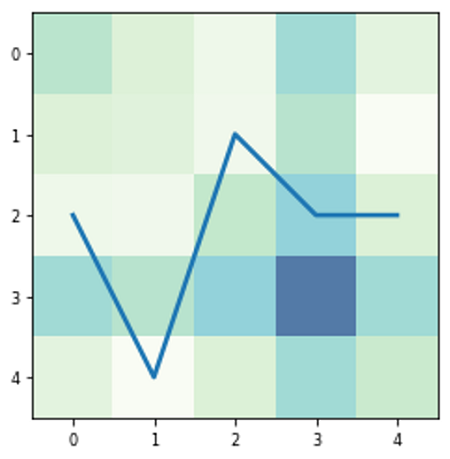# Python | Hybrid Line Plot with Heatmap

In this article, we are going to learn how to draw a line plot with a heatmap in the background for better data visualization?
Submitted by Anuj Singh, on August 08, 2020

Following example illustrates the implementation of our desired plot.## Python code for hybrid line plot with heatmap

```import numpy as np
import matplotlib.pyplot as plt

x = np.arange(5)
y = np.random.randint(1,5,5)
mata = np.random.random([5,4])
mataa = np.matmul(mata, mata.T)
plt.imshow(mataa, cmap='GnBu', alpha=0.7)
plt.plot(x,y, linewidth=2.5)
plt.axis(False)
plt.show()

plt.figure()
mata = np.random.random([5,4])
mataa = np.matmul(mata, mata.T)
plt.imshow(mataa, cmap='GnBu', alpha=0.7)
plt.plot(x,y, linewidth=2.5)
plt.show()
```

Output:

```Output is as Figure
```